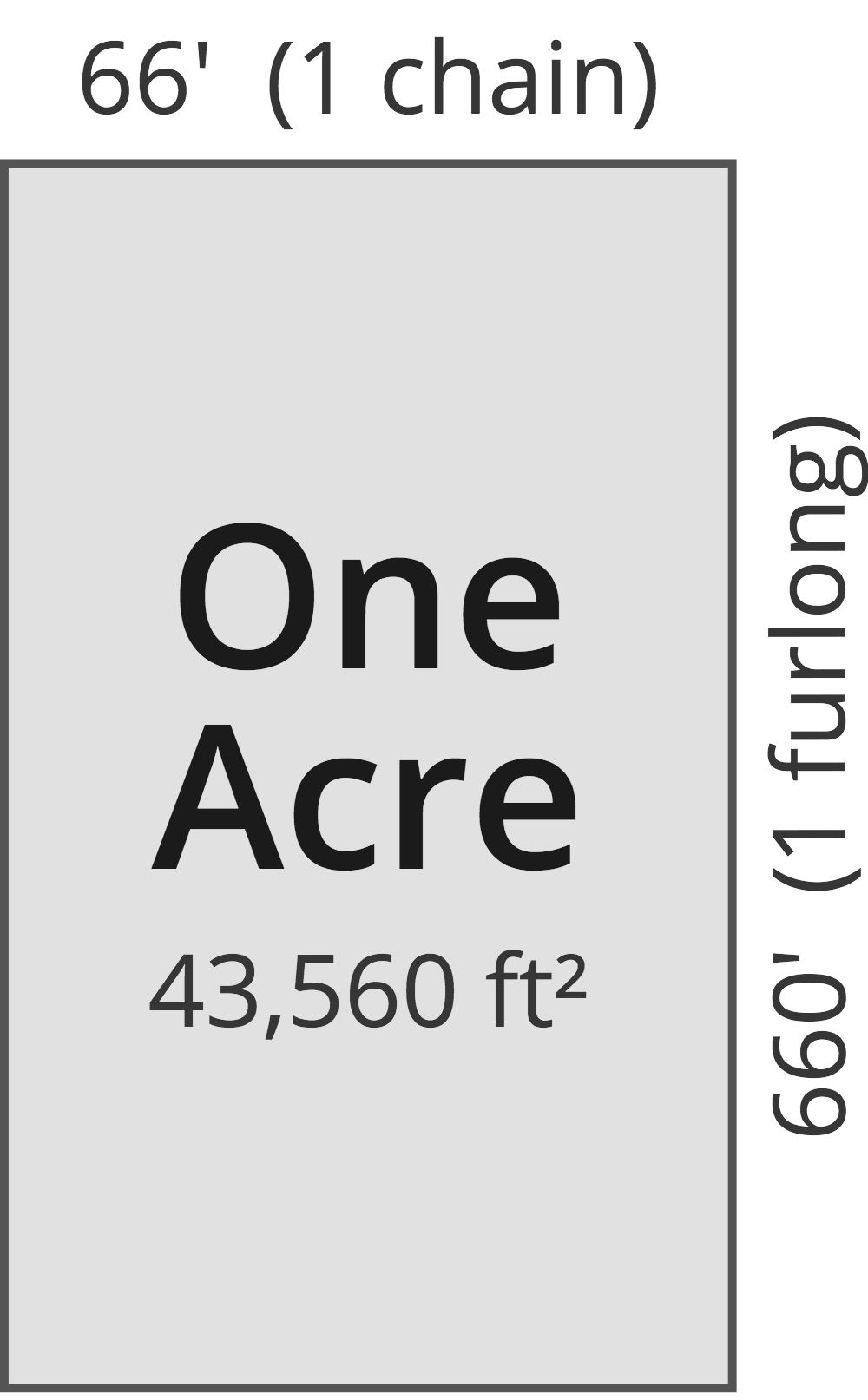# Acres to Square Yards Converter

Enter the area in acres below to get the value converted to square yards.

(find acres)
Results in Square Yards:1 ac = 4,840 sq yd

Do you want to convert square yards to acres?

## How to Convert Acres to Square Yards

To convert a measurement in acres to a measurement in square yards, multiply the area by the following conversion ratio: 4,840 square yards/acre.

Since one acre is equal to 4,840 square yards, you can use this simple formula to convert:

square yards = acres × 4,840

The area in square yards is equal to the area in acres multiplied by 4,840.

For example, here's how to convert 5 acres to square yards using the formula above.
square yards = (5 ac × 4,840) = 24,200 sq yd

### How Many Square Yards Are in an Acre?

There are 4,840 square yards in an acre, which is why we use this value in the formula above.

1 ac = 4,840 sq yd

## What is an Acre?

One acre is defined as the area equal to a space that is one chain (66 ft) by one furlong (660 ft), or 10 square chains. That's equal to 43,560 square feet or 1/640 of a square mile for those unfamiliar with those units of measure.The acre is a US customary and imperial unit of area. Acres can be abbreviated as ac; for example, 1 acre can be written as 1 ac.

You can use an acreage calculator to measure the area of a plot of land in acres by locating the boundaries on a map.

## What is a Square Yard?

One square yard is equivalent to the area of a square with sides that are each 1 yard in length. One square yard is roughly equal to 9 square feet or 0.836127 square meters.

The square yard is a US customary and imperial unit of area. A square yard is sometimes also referred to as a square yd. Square yards can be abbreviated as sq yd, and are also sometimes abbreviated as yd². For example, 1 square yard can be written as 1 sq yd or 1 yd².

You can use a square yards calculator to calculate the area of a space if you know its dimensions.

## Acre to Square Yard Conversion Table

Table showing various acre measurements converted to square yards.
Acres Square Yards
0.001 ac 4.84 sq yd
0.002 ac 9.68 sq yd
0.003 ac 14.52 sq yd
0.004 ac 19.36 sq yd
0.005 ac 24.2 sq yd
0.006 ac 29.04 sq yd
0.007 ac 33.88 sq yd
0.008 ac 38.72 sq yd
0.009 ac 43.56 sq yd
0.01 ac 48.4 sq yd
0.02 ac 96.8 sq yd
0.03 ac 145.2 sq yd
0.04 ac 193.6 sq yd
0.05 ac 242 sq yd
0.06 ac 290.4 sq yd
0.07 ac 338.8 sq yd
0.08 ac 387.2 sq yd
0.09 ac 435.6 sq yd
0.1 ac 484 sq yd
0.2 ac 968 sq yd
0.3 ac 1,452 sq yd
0.4 ac 1,936 sq yd
0.5 ac 2,420 sq yd
0.6 ac 2,904 sq yd
0.7 ac 3,388 sq yd
0.8 ac 3,872 sq yd
0.9 ac 4,356 sq yd
1 ac 4,840 sq yd

## References

1. National Institute of Standards and Technology, Specifications, Tolerances, and Other Technical Requirements for Weighing and Measuring Devices, Handbook 44 - 2019 Edition, https://nvlpubs.nist.gov/nistpubs/hb/2019/NIST.HB.44-2019.pdf